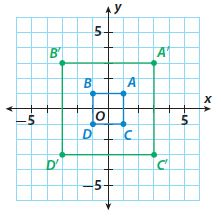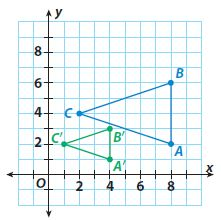Homework Explained - Math Practice 101Dear guest, you are not a registered member. As a guest, you only have read-only access to our books, tests and other practice materials.

As a registered member you can:

Registration is free and doesn't require any type of payment information. Click here to Register.
Go to page:
Chapter 10:Transformations and Similarity; Lesson 1: Properties of Dilations

Identify the scale factor used in each dilation.

• Question 17• Question 18### FOCUS ON HIGHER ORDER THINKING

• Question 19

Critical Thinking Explain how you can find the center of dilation of a triangle and its dilation.

• Type below:
• Question 20

Make a Conjecture

a. A square on the coordinate plane has vertices at (−2, 2), (2, 2), (2, −2), and (−2, −2). A dilation of the square has vertices at (−4, 4), (4, 4), (4, −4), and (−4, −4). Find the scale factor and the perimeter of each square.

•  Scale factor: Original perimeter: Image perimeter:
• Question 20

b. A square on the coordinate plane has vertices at (−3, 3), (3, 3), (3, −3), and (−3, −3). A dilation of the square has vertices at (−6, 6), (6, 6), (6, −6), and (−6, −6). Find the scale factor and the perimeter of each square.

•  Scale factor: Original perimeter: Image perimeter:
• Question 20

c. Make a conjecture about the relationship of the scale factor to the perimeter of a square and its image.

• Type below:

Yes, email page to my online tutor. (if you didn't add a tutor yet, you can add one here)